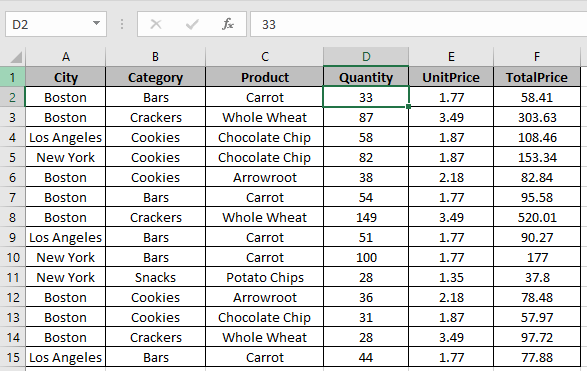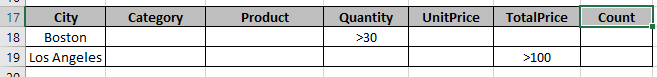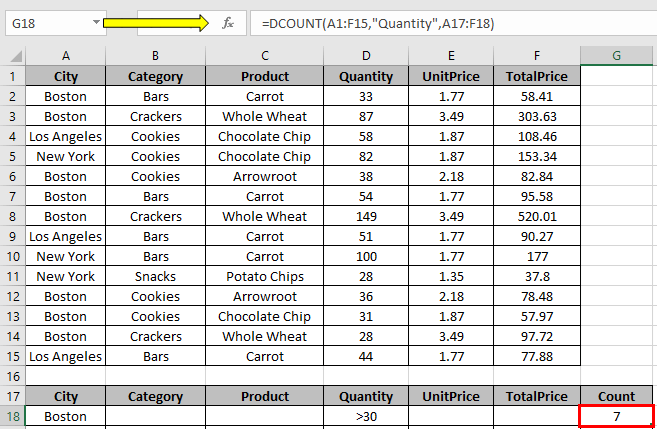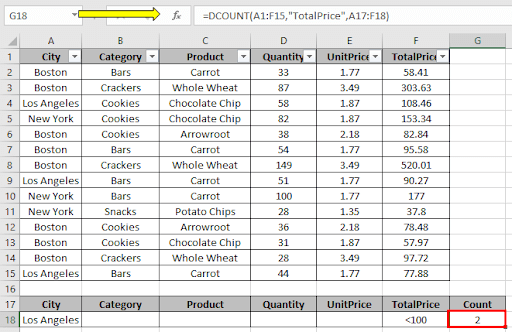# How to use the Excel DCOUNT function

In this article, we will learn about how to use the DCOUNT function in Excel.

The DCOUNT function in Excel returns the number of fields containing numeric values on a selected set of tables
Syntax:

=DCOUNT (database, [field], criteria)

database : including headers
field : matched header
criteria : including headers

Let’s understand this function using it an example.Here we have a table we need to count fields having certain condition.Use the formula to count fields having city “Boston” & “Quantity” greater than 30.

=DCOUNT(A1:F15,"Quantity",A17:F18)The count is 7.

Now

Use the formula to count fields having city “Los Angeles” & “TotalPrice” less than 100.

=DCOUNT(A1:F15,"TotalPrice",A17:F18)The count is 2.

Use the DCOUNTA function to count number fields having condition not only on numeric values.

Hope you understood how to use DCOUNT function in Excel. Explore more articles on Excel Count cells function here. Please feel free to state your query or feedback for the above article.

Related Article:

How to use the DAVERAGE Function in Excel

How to use the DMAX Function in Excel

How to use the DMIN Function in Excel

How to use the DSTDEV Function in Excel

How to use the DSTDEVP Function in Excel

How to use the DPRODUCT Function in Excel

How to use the DGET Function in Excel

Popular Articles:

How to use the VLOOKUP Function in Excel

How to use the COUNTIF function in Excel 2016

How to Use SUMIF Function in Excel

Terms and Conditions of use

The applications/code on this site are distributed as is and without warranties or liability. In no event shall the owner of the copyrights, or the authors of the applications/code be liable for any loss of profit, any problems or any damage resulting from the use or evaluation of the applications/code.